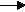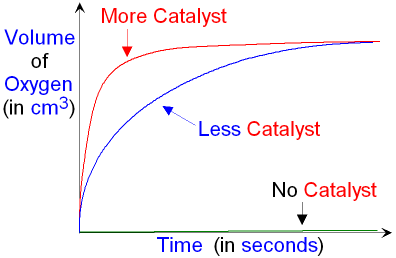gcsescience.com                                       14                                       gcsescience.com

Rates of Reaction

What is the Effect of using a Catalyst
for the Decomposition of Hydrogen Peroxide?

At room temperature hydrogen peroxide decomposes very slowly.
The presence of a catalyst may cause it to decompose quickly.

hydrogen peroxideoxygen   +   water.
2H2O2(aq)O2(g)   +   2H2O(l)

The rate of this reaction can be followed by
recording the volume of oxygen as it is produced
and plotting a graph of volume against time as
shown below. You must know a test for oxygen gas.

What is the Effect of using Different Amounts of Catalyst
for the Decomposition of Hydrogen Peroxide?

The catalyst used is manganese(IV) oxide - MnO2(s)
Using more catalyst will show an increase in reaction rate.
This is because more catalyst will have a
greater surface area for the reaction to take place.What is the Effect of using Different Catalysts
for the Decomposition of Hydrogen Peroxide?

The reaction can be performed using different catalysts to
compare how well each catalyst works for the same reaction.
A fair test must use the same amount of each catalyst.As you can see from the graph, manganese(IV) oxide (MnO2(s))
is the best catalyst for this reaction. The gradient of the
plot
for manganese(IV) oxide is greater (steeper) than the other two.
Copper(II) oxide (CuO(s)) is a less effective catalyst
than manganese(IV) oxide. Zinc oxide (ZnO(s)) is
the least effective of the three catalysts for this reaction.

gcsescience.com        The Periodic Table        Index        Catalyst Quiz        gcsescience.com